Hoeffding Dependence Coefficient

Subsections:

Hoeffding’s measure of dependence,
, is a nonparametric measure of association that detects more general departures from independence. The statistic approximates a weighted sum over observations of chi-square statistics for two-by-two classification tables (Hoeffding, 1948). Each set of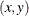values are cut points for the classification. The formula for Hoeffding’sis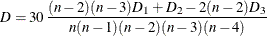where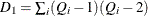,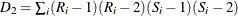, and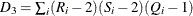.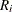is the rank of,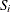is the rank of, and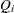(also called the bivariate rank) is 1 plus the number of points with bothand
values less than theth point.

A point that is tied on only thevalue orvalue contributes 1/2 toif the other value is less than the corresponding value for theth point.

A point that is tied on bothandcontributes 1/4 to. PROC CORR obtains thevalues by first ranking the data. The data are then double sorted by ranking observations according to values of the first variable and reranking the observations according to values of the second variable. Hoeffding’sstatistic is computed using the number of interchanges of the first variable. When no ties occur among data set observations, thestatistic values are between0.5 and 1, with 1 indicating complete dependence. However, when ties occur, thestatistic might result in a smaller value. That is, for a pair of variables with identical values, the Hoeffding’sstatistic might be less than 1. With a large number of ties in a small data set, thestatistic might be less than0.5. For more information about Hoeffding’s, see Hollander and Wolfe (1999).

Probability Values

The probability values for Hoeffding’sstatistic are computed using the asymptotic distribution computed by Blum, Kiefer, and Rosenblatt (1961). The formula is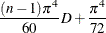which comes from the asymptotic distribution. If the sample size is less than 10, refer to the tables for the distribution ofin Hollander and Wolfe (1999).